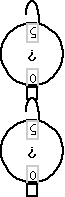Spring Scales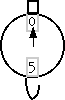This scale reads force in newtons.

Introduction

You can do some interesting investigations of forces using two spring scales.

Material

Two identical spring scales, like those used to weigh fish, or those found in physics class.

To Do and Notice

Have two people each hold one of the two spring scales.

Hook the two spring scales together. Notice how the scales are connected together below, rather than attaching them hook-to-hook, they are aligned hook to top. This makes it easier to compare the readings on both scales.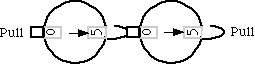Two spring scales hooked together horizontally always read the same force.

Have the people pull back and forth.

Notice that the spring scales always read the same value.

Trick question, what is the acceleration of each spring scale? What is the net force on the pair of spring scales?

The scales are always at rest and remain at rest, their acceleration is zero.
Thus, the net force on them is zero from Newton's Law F = m a. Where "a" is the acceleration, which here is zero, and F is the net force on the object.

Notice that scales do not read the net force of zero.

What's Going On?

Scales read the magnitude of one of the two forces acting on them,
the force they exert on the thing they are attached to.

So one scale reads the magnitude of the action force the other scale exerts on it.

The other scale reads the reaction force.

By Newton's third law the action and reaction forces are equal and opposite.

Thus, these forces always have the same magnitude and so the scales always read the same value.

Going Further

Hold one scale by its top handle with nothing attached to it. Notice the scale reads zero.Hold the scale by its top, the scale reads 0.

Turn the scale over. Hold the scale by the bottom hook that is meant to be attached to a fish. What do you predict it will read?

Notice that the scale reads ...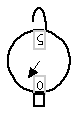Hold the scale by its bottom hook, upside down, it reads the weight of the scale.

The weight of the scale. It does not read zero. You pull up with the weight of the scale, gravity pulls down. the net force on the scale is zero but the scale reads one of the components of the net force.

Hold two scales vertically. Hold the top handle of one scale, hang the top of the second scale from the bottom of the first.

Predict what the scales will read.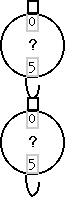Turn the two scale stack upside down. Hold both scales by the bottom hook of the top scale.